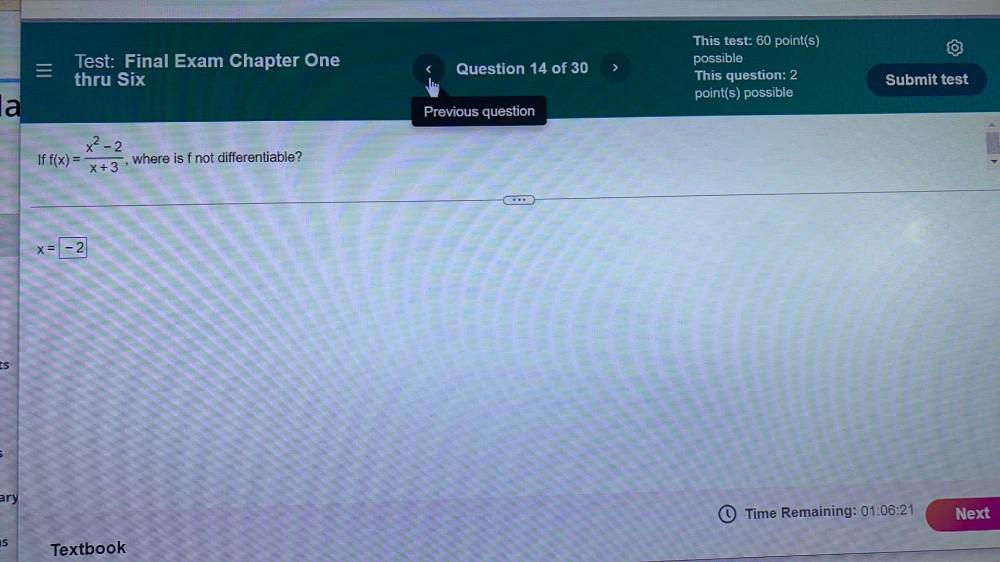Question:

# If f(x) = (x²-2)/(x+3) where is f not differentiable? X =

Last updated: 7/26/2022If f(x) = (x²-2)/(x+3) where is f not differentiable? X =